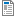Calculate Unit Price

Calculate Unit Price

Rating:
How are you using this activity? (0 comments)
Activity Description

The learner will calculate the cost of each item sold in a package.

Materials (optional):
• Pen/pencil
• Calculator (if needed)
Word File:CalculateUnitPrice_EI_C1.2.doc — Never downloaded
PDF - Practitioner's Version:CalculateUnitPrice_EI_C1.2.pdf — Never downloaded